Next: Theorem of Kutta and Up: Incompressible Aerodynamics Previous: Incompressible Aerodynamics

# Introduction

This chapter investigates the forces exerted on a stationary obstacle situated in a uniform, high Reynolds number, subsonic (and, therefore, effectively incompressible--see Section 1.17) wind, on the assumption that the obstacle is sufficiently streamlined that there is no appreciable separation of the boundary layer from its back surface. Such an obstacle is termed an airfoil (or aerofoil). Obviously, airfoil theory is fundamental to the theory of flight. Further information on this subject can be found in Milne-Thomson 1958.

The flow around an airfoil is essentially irrotational and inviscid everywhere apart from a thin boundary layer localized to its surface, and a thin wake emitted by its trailing edge. (See Sections 8.5 and 8.6.) It follows that, for the flow external to the boundary layer and wake, we can write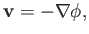(9.1)

which automatically ensures that the flow is irrotational. Assuming that the flow is also incompressible, so that, the velocity potential,, satisfies Laplace's equation: that is,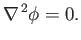(9.2)

The appropriate boundary condition at the surface of the airfoil is that the normal velocity be zero. In other words,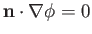, whereis a unit vector normal to the surface. In general, the tangential velocity at the airfoil surface, obtained by solving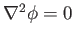in the external region, subject to the boundary conditionon the surface, is non-zero. Of course, this is inconsistent with the no slip condition, which demands that the tangential velocity be zero at the surface. (See Section 8.2.) However, as described in the previous chapter, this inconsistency is resolved by the boundary layer, across which the tangential velocity is effectively discontinuous, being non-zero on the outer edge of the layer (where it interfaces with the irrotational flow), and zero on the inner edge (where it interfaces with the airfoil). The discontinuity in the tangential velocity across the layer implies the presence of bound vortices covering the surface of the airfoil (see Section 9.7), and also gives rise to a friction drag acting on the airfoil in the direction of the external flow. However, the magnitude of this drag scales as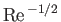, whereis the Reynolds number of the wind. (See Section 8.5.) Hence, such drag becomes negligibly small in the high Reynolds number limit. In the following, we shall assume that any form drag, due to the residual separation of the boundary layer at the back of the airfoil, is also negligibly small. Moreover, for the sake of simplicity, we shall initially restrict our discussion to two-dimensional situations in which a high Reynolds number wind flows transversely around a stationary airfoil of infinite length (in the-direction) and uniform cross-section (parallel to the-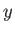plane).Next: Theorem of Kutta and Up: Incompressible Aerodynamics Previous: Incompressible Aerodynamics
Richard Fitzpatrick 2016-03-31